GPS Chips and Modules Information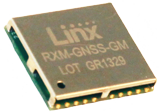GPS chips and modules provide users with instantaneous location and time data anywhere on Earth. The Global Positioning System (GPS) is a spaced-based navigational system that provides exact location and time information to any GPS receiver. The system uses many satellites (at least 24), at approximate orbits of 12,000 miles above the Earth, that transmit data using high frequency radio signals ranging from 1 to 1.5 GHz. The data is received by GPS receivers that process it to calculate time and location of the receiver. The array of satellites is positioned such that the sky above any location on Earth will always have at most 12 satellites.

The basic operation of GPS is as follows: signals transmitted from at least three satellites are used to calculate the position of the receiver on Earth (latitude and longitude), and a fourth satellite is used to calculate the altitude in respect to the surface of the Earth. Signals from the satellites include data on where in the sky the satellite is located (orbital data), and the time (timestamp) when the signal is sent. This data allows the computer program in the receiver to calculate the distance from the satellite.

The GPS provides two types of services, based on the level of clearance and security of the users:

Precise Positioning Service (PPS): This level of service is only available to the military of the United States and its allies, certain U.S. agencies, and few selected civil users. The users must have special PPS receivers with unique cryptographic algorithms and special keys. The accuracy of the PPS service is (as of this guide's publishing):

• Horizontal accuracy: 22 m
• Vertical accuracy: 27.7 m
• Time (UTC) accuracy: 200 ns

Standard Positioning Service (SPS): This level of service is designed to provide civil users with less accurate navigation than the PPS receivers. The level of accuracy allowed for SPS is as follows:

• Horizontal accuracy: 100 m
• Vertical accuracy: 156 m
• Time (UTC) accuracy: 340 ns

Even though the system is used by millions worldwide, the GPS is totally controlled, funded, and supported by the United States government and operated by United State Air Force (USAF). Since 1978, the date if its first launch, the GPS has provided positioning, navigation, and timing to million of users with civil or military receivers. The GPS provides these services to users anywhere in the world, in any weather, day or night. The system is an indispensable global utility that is essential in the development of economic growth, transportation, global economic infrastructure, and global security.

Measurements

The most important parameter the receiver should accurately calculate is the distance of each satellite from the receiver (on Earth). The distance is calculated using the velocity equation: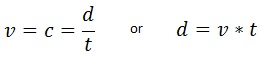In these equations (or c) is the speed of the object, d is the distance traveled during the travel time t.

For the case of the GPS, v is the speed of the radio wave from the satellite which is equal to the speed of light c, which is approximately: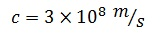In both the satellite and the receiver there is a clock. When the satellite sends a signal it includes the time. When the signal is received by the GPS receiver, it calculates the time traveled by the signal by subtracting the time the signal is received from the satellite timestamp. Travel time is calculated as follows: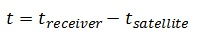And the distance from the satellite is calculated as follows: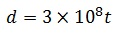Because the speed of light is quite large an effor of, say, 1 ms (1 x 10-6s), produces an error of 300 m (3 x 108) · (1 x10-6).

Therefore, it is clear the clock in the satellite and the clock in the receiver should be accurate and synchronized.

Accurate. Each satellite has an atomic clock that is extremely accurate. Even if the receiver clock is not an atomic clock (with the exception of certain military devices), the travel time can be calculated with good accuracy.

Synchronized. The synchronization of receiver and satellite clocks must also be extremely accurate, otherwise the travel time may be off by several milliseconds. Synchronization means that at any moment both clocks measure exactly the same time. Because the clock on Earth moves at the speed of Earth's orbital velocity and the clock on the satellite moves at much higher speed, Einstein's general relativity corrections should be made. Clocks in frames moving at different speeds undergo gravitational time dilation. If this dilation is not taken into account an error between the ground clock and the orbital clock of seconds per day is observed, where the satellite clock slows by that amount every day. This value gives an error in position of 11.7 km.

Therefore, adjustments should be made. Before sending the satellites to orbit, the satellite clock tick frequency is adjusted. This means that when the satellite is on Earth the two clocks are not synchronized, while when the satellite is in orbit the two clocks are synchronized. To understand better how general relativity applies to GPS technology, read the article Relativity in the Global Positioning System by Neil Ashby.

Trilateration

To locate the position of the receiver on Earth, first the distance of the receiver from three satellites needs to be determined. This process is called trilateration, a more complex version of triangulation. Triangulation techniques cannot be used with GPS; instead trilateration is used because four reference points are needed: latitude, longitude, altitude, and time.

Trilateration is a mathematical method that calculates position and time using several reference points. For this method to work, the GPS has to be able to clearly communicate with at least four satellites. In the jargon of GPS, this is called a lock or fix.

Start by determining the distance from the first satellite—this is the distance shown in Figure 1. This means that the location of the receiver is on some point on the surface of the sphere of radius centered at the first satellite.

With the knowledge of the distance from a second satellite, known as distance from a second satellite. Now, the receiver should be located also on the surface of the second sphere. The intersection of these two spheres is a circle, as shown in Figure 1. The location of the receiver can be any point on the circumference of this circle that contains an infinite number of points.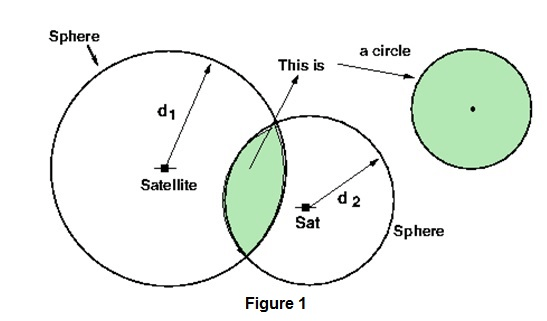To reduce the number of locations of the receiver, the distance from a third satellite is needed.This is depicted in Figure 2. The third satellite sphere will cut through the circle that is the intersection of the first two spheres. This will produce two points, a and b in the figure, as possible locations of the GPS receiver.To decide which of the two points is the true location of the GPS receiver, the signal from a fourth satellite can be used. This is normally not necessary because one of the two points will be too far away from the surface of the Earth, or the point is moving at a huge speed and it can be rejected.

With a fourth satellite, as seen in Figure 3, it is possible to determine the exact location. In the figure point b is the Earth location of the receiver.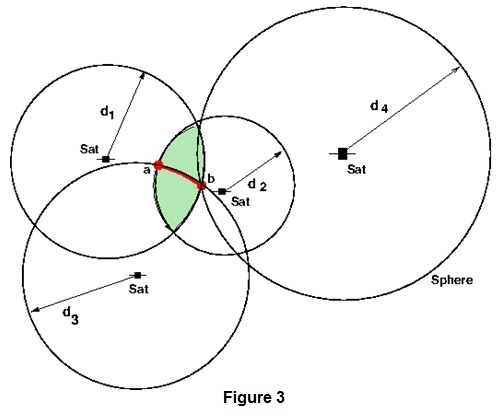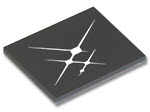Types

There are many types of GPS receivers, but from the form factor perspective they can be divided into two categories: integrated circuit (IC) chips and full modules. An IC GPS needs to be connected to a display, such as a computer or mobile device, among other peripherals, while a module is a complete unit with a display and any necessary peripherals.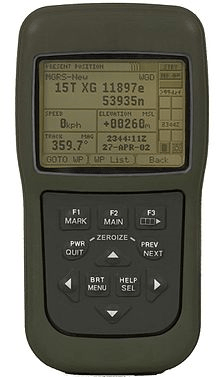Specifications

Supply Voltage. This is the operating voltage of the device. Exceeding the manufacturer recommendations can result in damaging the unit.

Interface. The GPS data can be retrieved from the receiver and sent to a processing device such a microcontroller or computer. The best type of interface for this activity is a serial port. The accompanying image shows how to connect the GPS unit to a microcontroller and to a computer. Other types of interfaces include Fibre Channel, I2C, PCI, RS232, RS422, and USB, among others.

Storage/memory capacity. This is where maps are stored in the GPS.

Bit rate. This the rate of transmission out of the GPS. Typical values are 90 kbps and 57.6 kbps.

Time to first fix (TTFF). This is the time that the GPS unit takes to fully compute the time and position of at least four satellites.

Update rate. This is the frequency that the unit calculates its position. The normal frequency is 1Hz, or once per second.

Standards

GPS Data

Data sent to the receiver from the satellite is in one of many message formats, but the standard used by most of receivers is the format specified by the National Marine Electronics Association standard NMEA 0183. This is the standard data format used by marine electronics devices such as sonar, autopilot, and GPS. NMEA messages sent to receivers include time, longitude, latitude, number of satellite fixes, and altitude.